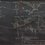# A weird questionI'm sure almost all of you are familiar with Ramanujan's number, 1729. What about 87539319, or 6963472309248?

Define the $n$th strict taxicab number $T^{*}(n)$ as the smallest number which can be expressed as the sum of two positive integral cubes in exactly $n$ ways. Then, it turns out that $T^{*}(3) = 87539319$ and $T^{*}(4) = 6963472309248$.

$\text{Is the sequence } \lbrace T^{*}(n) \rbrace_{n=1} \text{ strictly monotonically increasing?}$

Obviously (I think) this is not a question we can currently answer. I don't even know if the sequence is bounded above. Still, I think it's a pretty fun question to ask as a sort of time capsule, to look at how far the tools of mathematics will sharpen in the decades to come.Note by Jake Lai
6 years, 5 months ago

This discussion board is a place to discuss our Daily Challenges and the math and science related to those challenges. Explanations are more than just a solution — they should explain the steps and thinking strategies that you used to obtain the solution. Comments should further the discussion of math and science.

When posting on Brilliant:

• Use the emojis to react to an explanation, whether you're congratulating a job well done , or just really confused .
• Ask specific questions about the challenge or the steps in somebody's explanation. Well-posed questions can add a lot to the discussion, but posting "I don't understand!" doesn't help anyone.
• Try to contribute something new to the discussion, whether it is an extension, generalization or other idea related to the challenge.

MarkdownAppears as
*italics* or _italics_ italics
**bold** or __bold__ bold
- bulleted- list
• bulleted
• list
1. numbered2. list
1. numbered
2. list
Note: you must add a full line of space before and after lists for them to show up correctly
paragraph 1paragraph 2

paragraph 1

paragraph 2

[example link](https://brilliant.org)example link
> This is a quote
This is a quote
    # I indented these lines
# 4 spaces, and now they show
# up as a code block.

print "hello world"
# I indented these lines
# 4 spaces, and now they show
# up as a code block.

print "hello world"
MathAppears as
Remember to wrap math in $$ ... $$ or $ ... $ to ensure proper formatting.
2 \times 3 $2 \times 3$
2^{34} $2^{34}$
a_{i-1} $a_{i-1}$
\frac{2}{3} $\frac{2}{3}$
\sqrt{2} $\sqrt{2}$
\sum_{i=1}^3 $\sum_{i=1}^3$
\sin \theta $\sin \theta$
\boxed{123} $\boxed{123}$

Sort by:

How did you derive $T^{*}(3)$ and $T^{*}(4)$?

- 6 years, 5 months ago

nvm... heres the article

- 6 years, 5 months ago

Actually, the regular (nonstrict) taxicab numbers $T(n)$ is the most common definition. For small $n$ it corresponds to $T^{*}(n)$. I was thinking about whether or not it was possible if $T^{*}(n) > T^{*}(n+1)$ for large $n$ despite the latter being expressible in one more way than the other.

- 6 years, 5 months ago